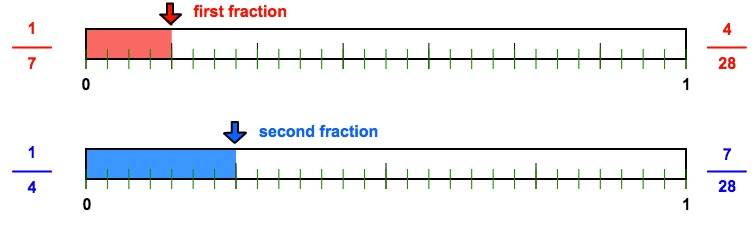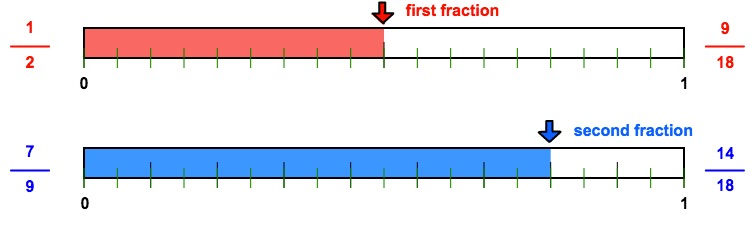# RATL 2 | Lesson 1 | Explore (Rational Numbers)Solution

Review of rational numbers.

1. Order the numbers below from least to greatest.

$$\dfrac{5}{12} \hspace{5mm} \dfrac{3}{8} \hspace{5mm} \dfrac{1}{7} \hspace{5mm} \dfrac{1}{2} \hspace{5mm} \dfrac{7}{9} \hspace{5mm}$$$$0.25 \hspace{5mm} 0.333$$

1. How can you be sure you have the correct order? Use pictures, diagrams, and other strategies to explain your thinking.Note you could also find the LCD, rename the fractions and then compare the numerators.

The fractions and decimals above are examples of rational numbers.